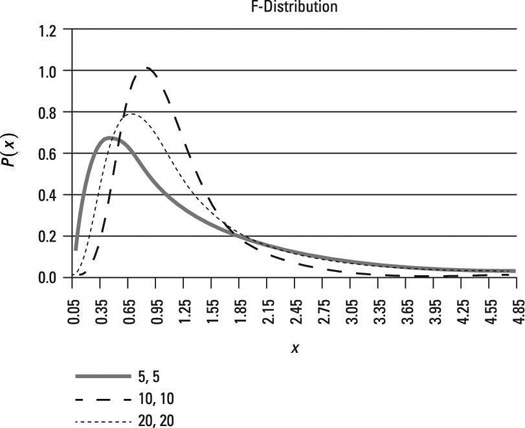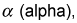The F-distribution is a continuous probability distribution, which means that it is defined for an infinite number of different values. The F-distribution can be used for several types of applications, including testing hypotheses about the equality of two population variances and testing the validity of a multiple regression equation.

The F-distribution shares one important property with the Student's t-distribution: Probabilities are determined by a concept known as degrees of freedom. Unlike the Student's t-distribution, the F-distribution is characterized by two different types of degrees of freedom — numerator and denominator degrees of freedom.

The F-distribution has two important properties:

• It's defined only for positive values.

A distribution is positively skewed if the mean is greater than the median. (The mean is the average value of a distribution, and the median is the midpoint; half of the values in the distribution are below the median, and half are above.)

A good example of a positively skewed distribution is household incomes. Suppose that half of the households in a country have incomes below \$50,000 and half have incomes above \$50,000; this indicates that the median household income is \$50,000. Among households with incomes below \$50,000, the smallest possible value is \$0. Among households with incomes above \$50,000, there may be incomes of several million dollars per year. This imbalance between incomes below the median and above the median causes the mean to be substantially higher than the median. Suppose for example that the mean income in this case is \$120,000. This shows that the distribution of household incomes is positively skewed.The shape of the F-distribution varies with its degrees of freedom (df).

This figure shows a graph of the F-distribution for different combinations of numerator and denominator degrees of freedom. In each case, numerator degrees of freedom are listed first, and denominator degrees of freedom are listed second. The level of significance in each case is 0.05.

A level of significance is used to test a hypothesis. A hypothesis test begins with a null hypothesis; this is a statement that's assumed to be true unless there is strong contrary evidence. There is also an alternative hypothesis; this is a statement that is accepted in place of the null hypothesis if there's sufficient evidence to reject the null hypothesis.

The level of significance, designatedrefers to the probability of incorrectly rejecting the null hypothesis when it is actually true. This is known as a Type I error. By contrast, a Type II error occurs when you fail to reject the null hypothesis when it's actually false. Therefore, with a level of significance of 0.05, there is a 5 percent chance of committing a Type I error.

The figure shows that the distribution isn't defined for negative values (as you can see, no negative values appear along the horizontal axis). Additionally, as the number of degrees of freedom increases, the shape of the distribution shifts to the right. The distribution has a long right tail (more formally, it's skewed to the right, or positively skewed).